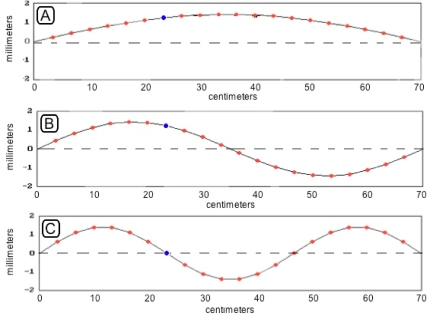# A120506030centimeters104070B102030centimeters40506070C30102040506070centimetersmillimetersmillimetersmillimeters

Question
30 views

In the figure at the right is shown three graphs of the shapes of the same taut elastic string in three different circumstances, labeled A, B, and C. In some of the problems below, the string represents a guitar string, tied down at both ends, while in others, it is part of a very long telephone wire whose ends are not shown. Be careful to note which is which for each problem! The string is light enough that the effects of gravity can be ignored. In each case, the bit of string at the position x = 23 cm is painted blue.

1. Suppose the three graphs all represent the same string, tied down at x = 0 and x = 70 cm, and at the same tension. What can you say about the three frequencies of oscillation?

a. Situation A has the highest frequency.
b. Situation B has the highest frequency.
c. Situation C has the highest frequency.
d. The three frequencies are all the same.
e. You cant say anything from the information given.

2. Suppose the three graphs all represent the same string, tied down at x = 0 and x = 70 cm, but their tensions have been adjusted so that all three situations are all oscillating at the same frequency. What can you say about the tensions in the three cases?

a. You cant adjust the tensions to make the frequencies the same since the wavelengths are different.
b. The tension in string C is the greatest.
c. The tension is string A is the greatest.
d. All three tensions are the same.
e. You cant say anything from the information given.

3. Suppose that graph C represents the string, tied down at x = 0 and x = 70 cm, under tension. What can you say about the acceleration of the blue bead in case C at the instant shown?

a. It is upward.
b. It is downward.
c. It is 0.
d. You cant say anything from the information given.help_outlineImage TranscriptioncloseA 1 20 50 60 30 centimeters 10 40 70 B 10 20 30 centimeters 40 50 60 70 C 30 10 20 40 50 60 70 centimeters millimeters millimeters millimeters fullscreen
check_circle

Step 1

(A) Situation A is a fundamental mode of vibration while situation B and C are second and third harmonic. So the frequency is higher for the situation C. option (B) is correct.

Step 2

(b) if frequency , length and linear density are same for three graphs then the tensions are also directly proportional . so tension in the situation C is greater than other two. As speed is constant frequency is inverse...

### Want to see the full answer?

See Solution

#### Want to see this answer and more?

Solutions are written by subject experts who are available 24/7. Questions are typically answered within 1 hour.*

See Solution
*Response times may vary by subject and question.
Tagged in

### Wave Motion